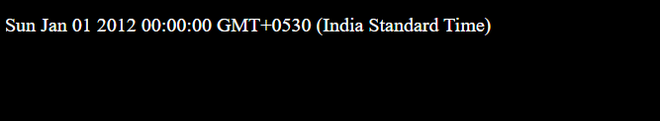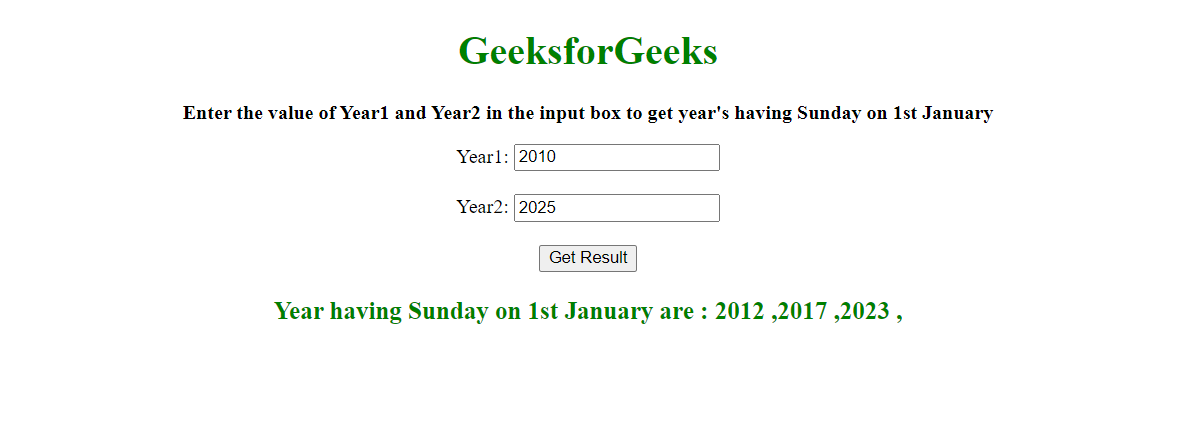# Find 1st January be Sunday between a range of years in JavaScript ?

• Difficulty Level : Easy
• Last Updated : 10 Dec, 2020

Given a range of years, the task is to find the year having Sunday on 1st January.

Approach: We can use javaScript Dates to get the years having Sunday on 1st January. In JavaScript, Date objects are based on a time value that is the number of milliseconds since 1 January 1970 UTC.

We can declare Date object in the following way:

In this program, we will use the last one. The new Date(year, month, day) will return the day, month, and year of the parameter given.

Example:

## Javascript

 `// Creating date object ` `// (Year , month , Day) ` `var` `A = ``new` `Date(2012, 0, 1); ` ` `  `// Printing the date ` `document.write(A);`

Output:Find 1st January be a Sunday between a range of years using JavaScript?

After the creation of the Date object with given parameters, we can access the day of the week 0(Sunday) to 6(Saturday) from the getDay() function.

The getDay() method is used to get the day of the week for the specified date according to local time, where 0 represents Sunday.

Source Code:

## HTML

 ` ` `<``html``> ` ` `  `<``head``> ` `    ``<``style``> ` `        ``body { ` `            ``text-align: center; ` `        ``} ` ` `  `        ``h1 { ` `            ``color: green; ` `        ``} ` ` `  `        ``#geeks { ` `            ``font-size: 16px; ` `            ``font-weight: bold; ` `        ``} ` ` `  `        ``#gfg { ` `            ``color: green; ` `            ``font-size: 20px; ` `            ``font-weight: bold; ` `        ``} ` `    `` ` ` ` ` `  `<``body``> ` `    ``<``h1``>GeeksforGeeks ` `    ``<``p` `id``=``"geeks"``> ` ` `  ` `  `    ``<``label` `for``=``"Year1"``>Year1: ` `    ``<``input` `type``=``"number"` `id``=``"year1"`  `        ``name``=``"Year1"``><``br``><``br``> ` ` `  `    ``<``label` `for``=``"Year2"``>Year2: ` `    ``<``input` `type``=``"number"` `id``=``"year2"`  `        ``name``=``"Year2"``><``br``><``br``> ` ` `  `    ``<``button` `onClick``=``"GFG_Fun()"``> ` `        ``Get Result ` `    `` ` `    ``<``p` `id``=``"gfg"``> ` ` `  ` `  `    ``<``script``> ` `        ``let s = `Enter the value of Year1  ` `        ``and Year2 in the input box to get  ` `        ``year's having Sunday on 1st January`; ` ` `  `        ``document.getElementById("geeks") ` `            ``.innerHTML = `<``p``>\${s}`; ` ` `  `        ``function GFG_Fun() { ` `            ``var y1 = Number(document ` `                ``.getElementById('year1').value); ` ` `  `            ``var y2 = Number(document ` `                ``.getElementById('year2').value); ` ` `  `            ``var res = " "; ` `             `  `            ``for (let year = y1; year <= y2; ++year) { ` `                ``const c_year = new Date(year, 0, 1); ` `                ``if (c_year.getDay() === 0) { ` `                    ``res += year + " ,"; ` `                ``} ` `            ``} ` ` `  `            ``if (y1 > y2) { ` `                ``document.getElementById('gfg').innerHTML ` `                     ``= "Year2 must be greater than Year1"; ` `            ``} else { ` `                ``if (res === " ") { ` `                    ``document.getElementById('gfg') ` `                    ``.innerHTML = `<``p``>No Year Exist`; ` `                ``} else { ` `                    ``document.getElementById('gfg') ` `                    ``.innerHTML = `<``p``> Year having  ` `                    ``Sunday on 1st January are :\${res}`; ` `                ``} ` `            ``} ` `        ``}  ` `    `` ` ` ` ` `  ` `

Output:My Personal Notes arrow_drop_up
Recommended Articles
Page :